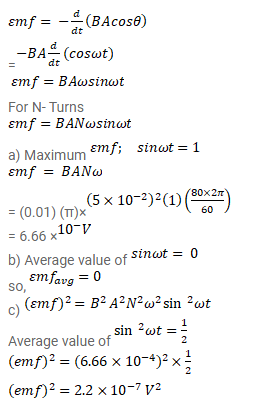# A circular coil of one turn of radius

Question:

A circular coil of one turn of radius $5.0 \mathrm{~cm}$ is rotated about a diameter with a constant angular speed of 80 revolutions per minute. A uniform magnetic field $B=0.010 \mathrm{~T}$ exists in a direction perpendicular at the axis of rotation. Find (a) the maximum emf induced by the average emf induced in the coil over a long period and (b) the average of the squares of emf induced over a long period.

Solution: